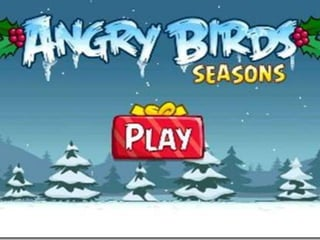Successfully reported this slideshow.

# Algebraic expression

39

Share×
1 of 29
1 of 29

# Algebraic expression

39

Share

## More Related Content

### Related Books

Free with a 14 day trial from Scribd

See all

### Related Audiobooks

Free with a 14 day trial from Scribd

See all

### Algebraic expression

1. 1. EXPRESS ME ALGEBRAICALLY!!<br />
2. 2.
3. 3. ALGEBRA<br />
4. 4. Find the definition of the following terms:<br />Algebra<br />variable<br />replacement set<br />substitution<br />constant<br />symbols of grouping<br />symbols of relationship<br />algebraic term<br />algebraic expression<br />factors<br />numerical coefficient<br />literal coefficient<br /> <br />
5. 5. ALGEBRA<br /><ul><li>Defined as a branch of mathematics which generalizes the facts in arithmetic.
6. 6. In the language of algebra, letters along with numbers and operation symbols are used to create expressions.</li></li></ul><li>VARIABLE<br /><ul><li>Can stand for different numbers at different times.
7. 7. Represents any number from a given replacement set.</li></li></ul><li>EXAMPLES:<br /><ul><li>Any letter in the ENGLISH ALPHABET such as X, Y, Z, A, B, and C.</li></ul>x + 7<br />b2 – 4ac<br />
8. 8. REPLACEMENT SET<br /><ul><li>The set of values of the variable.</li></li></ul><li>SUBSTITUTION<br /><ul><li>Replacing a variable by a constant.</li></li></ul><li>EXAMPLES:<br /><ul><li>let x ={1, 2, 3} replacement set</li></ul>x + 7<br />x= 1 x = 2 x = 3<br />x + 7 x + 7 x + 7<br />1 + 7 2 + 7 3 +7<br /> 8 9 10<br />
9. 9. CONSTANT<br /><ul><li>A symbol which has exactly one number in its replacement set.</li></li></ul><li>EXAMPLES:<br /><ul><li>7, 4, and 1 1.
10. 10. = 3. 141592654…</li></li></ul><li>SYMBOLS OF GROUPING<br />Parentheses: ( )<br />Brackets: [ ]<br />Braces: { }<br />Bar or fraction bar: ____<br />
11. 11. EXAMPLES:<br />1 1 – { 1 2 – [ 4 – (8 + 9 ) ] – 1 3 }<br />1 1 – { 1 2 – [ 4 – 1 7 ] – 1 3 }<br />1 1 – { 1 2 – 1 3 + 1 3 }<br />1 1 – 1 2<br />- 1<br />
12. 12. SYMBOLS OF RELATIONSHIP<br />≠ Is not equal to<br />≤ Is less than or equal to<br />≥ Is greater than or equal to<br />< Is less than<br />> Is greater than<br />= Equals, is equal to, is<br />
13. 13. EXAMPLES:<br />x + 7 < 1 0 <br />x = 1<br />1 + 7 < 1 0<br />8 < 1 0 √<br />
14. 14. FACTORS<br /><ul><li>The numbers and symbols in a product.</li></li></ul><li>EXAMPLES:<br />(-3) (2) = -6<br /> Factors product<br />(-3 x ) (2 x ) = -6 x2<br /> Factors product<br />
15. 15. NUMERICAL COEFFICIENT<br /><ul><li>The number in an algebraic term.</li></li></ul><li>LITERAL COEFFICIENT<br /><ul><li>A letter used to represent a number</li></li></ul><li>EXAMPLES:<br />4 x<br />Numerical coefficient Literal coefficient<br /><ul><li>45 abc </li></ul>Numerical coefficient Literal coefficient<br />
16. 16. ALGEBRAIC TERM<br /><ul><li>Either a single number, a letter, or a product of several numbers or letters.</li></li></ul><li>EXAMPLES:<br />x + 7<br />algebraic term term<br />2x + 3y – 4z <br />term term term<br />
17. 17. ALGEBRAIC EXPRESSION<br /><ul><li>A statement containing one or more terms connected by plus or minus signs. </li></li></ul><li>EXAMPLES:<br />x + 7<br />Algebraic expression<br />2x + 3y – 4z <br />expression<br />
18. 18. ALGEBRAIC EQUATION<br /><ul><li>A statement containing one or more terms connected by plus or minus signs with the symbol of equality. </li></li></ul><li>EXAMPLES:<br />x + 7 = 10<br />Algebraic Equation<br />2x + 3y – 4z = 20 <br />Equation<br />
19. 19. ALGEBRAIC term expression equation<br />2x, 29 2x + 29 2x + 29 = 50<br />x, y x - y x - y = -10<br />a2 , 2b, 5 a2 + 2b - 5 a2 + 2b – 5 = 9 <br />
20. 20. ASSIGNMENT<br /><ul><li> E-Math Book, page 165, Vocabulary and Concepts no.s 1-10.
21. 21. Page 167, VII: Identifying literal coefficients, no.s 59-63 and page 165, IX: Identifying numerical coefficients, no.s 65-69. </li></ul> <br />